### Home > CALC > Chapter 5 > Lesson 5.3.3 > Problem5-132

5-132.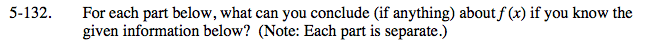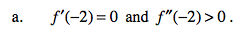This is a justification about whether f(x) has a local max or a local min at x = −2. But which one?

2nd Derivative Test.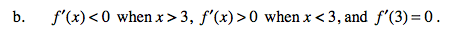This is a justification about whether f(x) has a local max or a local min at x = 3. But which one?

1st Derivative Test.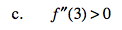Complete the sentence: At x = 3, the 2nd derivative is positive so f(3) is ____________________________

Two words.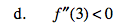Refer to hint in part (c).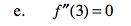There might be a point of inflection on f(x) at x = 3. But we do not know for sure. There are two ways to find out:
1. You could check the 2nd-derivative to the left of x = 3. If it changes signs then f(3) is a POI, if not then it's not.
2. You could evaluate the 3rd-derivative at x = 3. If it is NOT zero, then f(3) is a POI. If is is zero, then this method is inconclusive.
Since we are not given any extra information, we can only say that f(3) is a CANDIDATE for a point of inflection.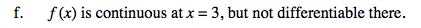Examples of points of NON-differentiability include: cusps, endpoints, jumps, holes and vertical tangents.
Which of the above attributes still possess continuity?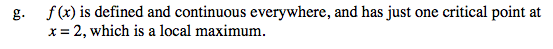Condition 1: Either f '(2) = 0 or f '(2) does not exist.
Condition 2: Refer to hints in part (a) or part (b).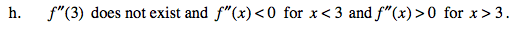Refer to hint in part (e).